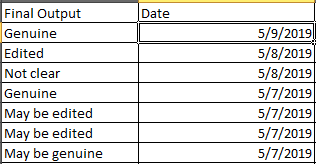# Comparing the excel cells and make a count

Hi,

I want to compare the excel cells from date column and write the count.
Ex:- If date is match then read the output column and make the entry in sheet2.

Basically I want to update the sheet 2 with date wise record.

Please find the attached copy of excel:-
Book1.xlsx (45.3 KB)

I have read lot of forum articles, actually I am confuse when date will change then how we can make the records.

Thanks you in advance.
@kirti.iyer @ClaytonM

Apologies, if I use too much .net coding here, but I find that it sometimes streamlines the process. And, this is kind of how I would end up doing this with my current thinking.

So we have this data:…with the main dataset being the Date column

This is also assuming that when you add dates to this dataset, they only go in the Date column.

So first thing to do, I believe, is that you need to be able to identify which column each output value goes to. To do so, you can create 2 array variables: one for the sheet2 columns and one for the values associated with them.

``````outputColumns = {"Number of Genuine","Number of Edited","Number of Not Clear","Number of May be genuine","Number of May be edited"}
outputValues = {"Genuine","Edited","Not clear","May be genuine","May be edited"}
outputDateColumn = "Date"
outputTotalColumn = "Total Count"
inputValueColumn = "Final Output"
inputDateColumn = "Date"
totalCount <Of Int32> //count of each date
totals <Of Int32()> //counts array of each outputValue
``````

So, essentially, you can loop through each unique date in the data, calculate the total count, then use the array variables to loop for each value and calculate its count.

Here is an example psuedocode to represent the steps and .net coding, where inputData is the dataset:

``````Build Data Table with 7 columns for output //where outputData is Data Table
Rename columns with For Each activity: ForEach col In outputData //TypeArgument is DataColumn and use output index
Assign: col.ColumnName = outputColumns(index)

For Each activity: ForEach d In inputData.AsEnumerable.Select(Function(r) Convert.ToDate(r(inputDateColumn).ToString.Trim) ).ToArray.Distinct //TypeArgument is String
Assign: rowsPerDate = inputData.AsEnumerable.Where(Function(r) Convert.ToDate(r(inputDateColumn).ToString.Trim) = d ).ToArray
Assign: totalCount = rowsPerDate.Count
Assign: totals = outputValues.Select(Function(x) 0).ToArray //initialize totals array to zeros

For Each activity: ForEach v In outputValues //use output index
Assign: totals(index) = rowsPerDate.Where(Function(r) r(inputValueColumn).ToString.Trim.ToUpper.Equals(v.Trim.ToUpper) ).ToArray.Count

Add Data Row: ArrayRow Property set to {d.ToString("MM/dd/yyyy")}.Concat({totalCount}).Concat(totals).ToArray

Write Range with outputData
``````

Hopefully, that is not too confusing.
To rehash:
—Create output data table
—Loop through distinct dates
------Assign total count for each date
------Initialize totals array to all zeros
------Loop through each value for the date
---------Assign total for each value of the date
------Concatenate each total to an array to be used with Add Data Row
—Write output data to new sheet

Regards.

1 Like